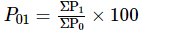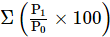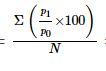# Sandeep Garg Solutions Class 11 Economics Chapter 9 - Index Numbers

Sandeep Garg Class 11 Economics Solutions Economics Chapter 9 – Index Numbers are prepared by professional economics educators from the contemporary edition of Sandeep Garg Economics Class 11 textbook solutions.

We at BYJU’S provide Sandeep Garg Economics Class 11 Solutions to give a comprehensive insight into the subject to the students. These insights will be a valuable advantage to students while completing their homework or while studying for their exams.

Here are some questions with solutions on Index numbers.

## Sandeep Garg Solutions Class 11 – Chapter 9

Question 1

Arrange an index number for the year 2015 on the basis of 2010 using the simple aggregative method:

 Goods Unit Cost 2010 2015 Potato Kilo 100 500 Tomato Kilo 400 250 Onion Kilo 200 400 Oil litre 4 5 Fabric meter 2 3

 Goods 2010 Price (P0) 2015 Price (P1) Potato Tomato Onion Oil Fabric 100 400 200 4 2 500 250 400 5 3 ΣP0 = 702 ΣP1 = 928

P01 =

$$\begin{array}{l}\frac{\sum P_{1}}{\sum P_{0}}\, X\, 100\end{array}$$

P01 =

$$\begin{array}{l}\frac{928}{702}\, X\, 100\end{array}$$

P01 = 132

Question 2

Form an index number using 2010 price:

 Items P Q R S T U Prices (2011) 220 50 370 110 70 90 Prices (2016) 250 80 500 130 72 100

 Items Price in 2011 (P0) Price in 2016 (P1) P Q R S T U 220 50 370 110 70 90 250 80 500 130 72 100 ΣP0 = 910 ΣP1 = 1152

P01 =

$$\begin{array}{l}\frac{\sum P_{1}}{\sum P_{0}}\, X\, 100\end{array}$$

P01 =

$$\begin{array}{l}\frac{1152}{910}\, X\, 100\end{array}$$

P01 = 126.59

Question 3

Prepare an index number for 2014 on the basis of 2010 from the below data using aggregate expenditure method.

 Particulars Quantity (kg.) Prices 2010 2014 G 9 21 25 H 6 20 22 I 4 19 23 J 3 9 10 K 3 5 9

 Article 2010 Price (P0) 2014 Price (P1) Quantity (Q0) P0Q0 P1Q0 G H I J K 21 20 19 9 5 25 22 23 10 9 9 6 4 3 3 189 120 76 27 15 225 132 92 30 27 ΣP0Q0 = 427 ΣP1Q0 = 506

The consumer price index for the year 2014 is

$$\begin{array}{l}\frac{\sum P_{1}Q_{0}}{\sum P_{0}Q_{0}}\,\, \times 100\end{array}$$

=

$$\begin{array}{l}\frac{506}{427}\, X\, 100\end{array}$$

= 118.50

Question 4

Calculate the consumer index number by using the family budget method.

 Items Quantity Base Price Year Current Price Year Rent 45 200 225 Food 20 300 350 Clothing 8 150 175 Fuel 10 100 110 Misc. 17 200 250

 Items Base Price Year (P0) Current Price Year (P1) $$\begin{array}{l}I \,=\, \frac{P_{1}}{P_{0}} \, \times \, 100\end{array}$$ Quantity (Q0) P0Q0 (W) RW Rent 200 225 112.5 45 9000 1012500 Food 300 350 116.66 20 6000 699960 Clothing 150 175 116.66 8 1200 139992 Fuel 100 110 110 10 1000 110000 Misc. 200 250 125 17 3400 425000 ΣW= 20600 ΣWI = 2387452

The consumer price index for the current year as per family budget method is:

$$\begin{array}{l}\frac{\sum WI}{\sum W} = \frac{2387452}{20600} = 115.89\end{array}$$

Question 5

Arrange an index for 2015 taking 2010 using a simple aggregate and price relatives method

 Items A B C D Year 2010 Price 20 40 50 70 Year 2015 Price 30 50 70 90

Simple Aggregate Method

 Items 2010 Price (P0) 2015 Price (P1) A B C D 20 40 50 70 30 50 70 90 ΣP0 = 180 ΣP1 = 240=  240/180 × 100

= 133.33

Price Relative Method

 Items Year 2010 Price (P0) Year 2015 Price (P1) $$\begin{array}{l}R \,=\, \frac{P_{1}}{P_{0}} \, \times \, 100\end{array}$$ A 20 30 150 B 40 50 125 C 50 70 140 D 70 90 128.57 N= 4= 543.57

Simple Average of Price RelativesP01 = 543.57/ 4

= 135.89

The above-provided solutions are considered to be the best solution for ‘Sandeep Garg Economics Class 11 Solutions Chapter 9 – Index Numbers. Stay tuned to BYJU’S to learn more.